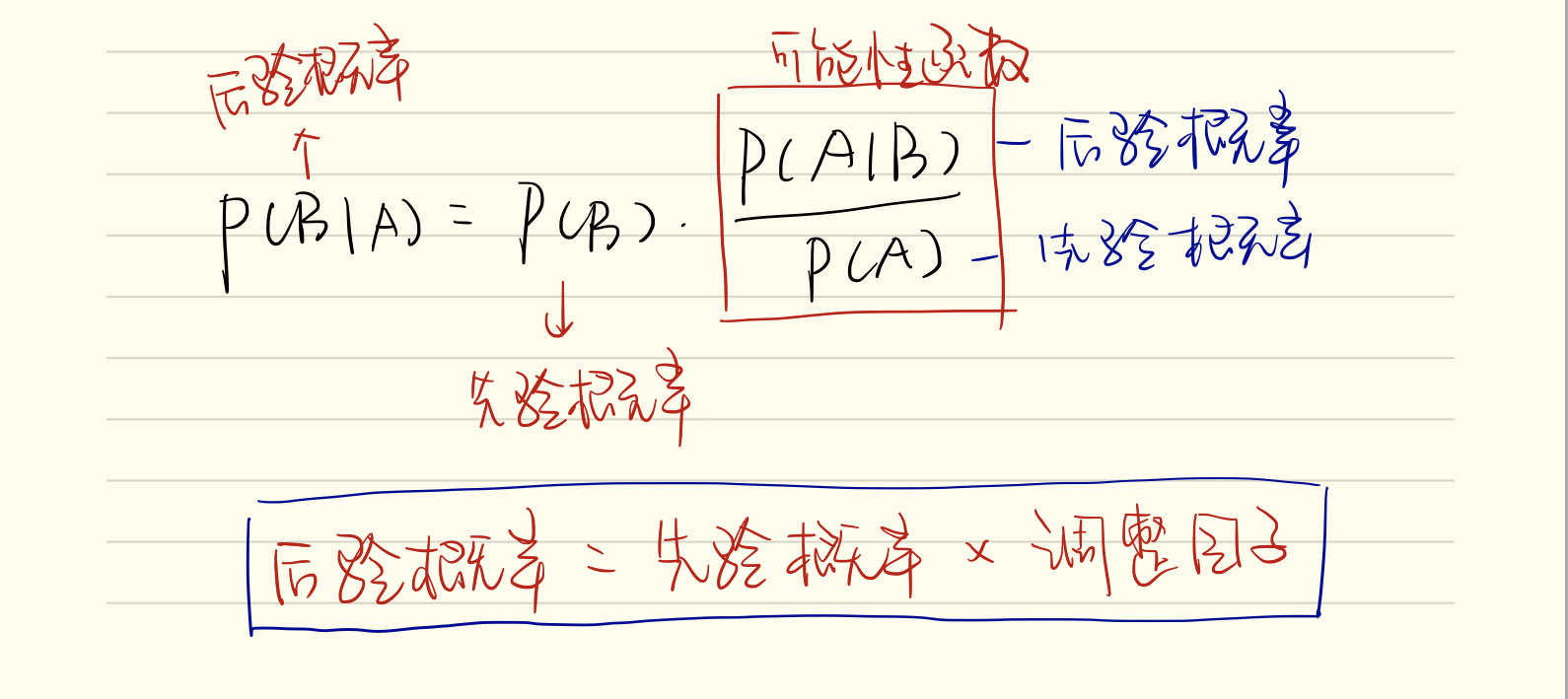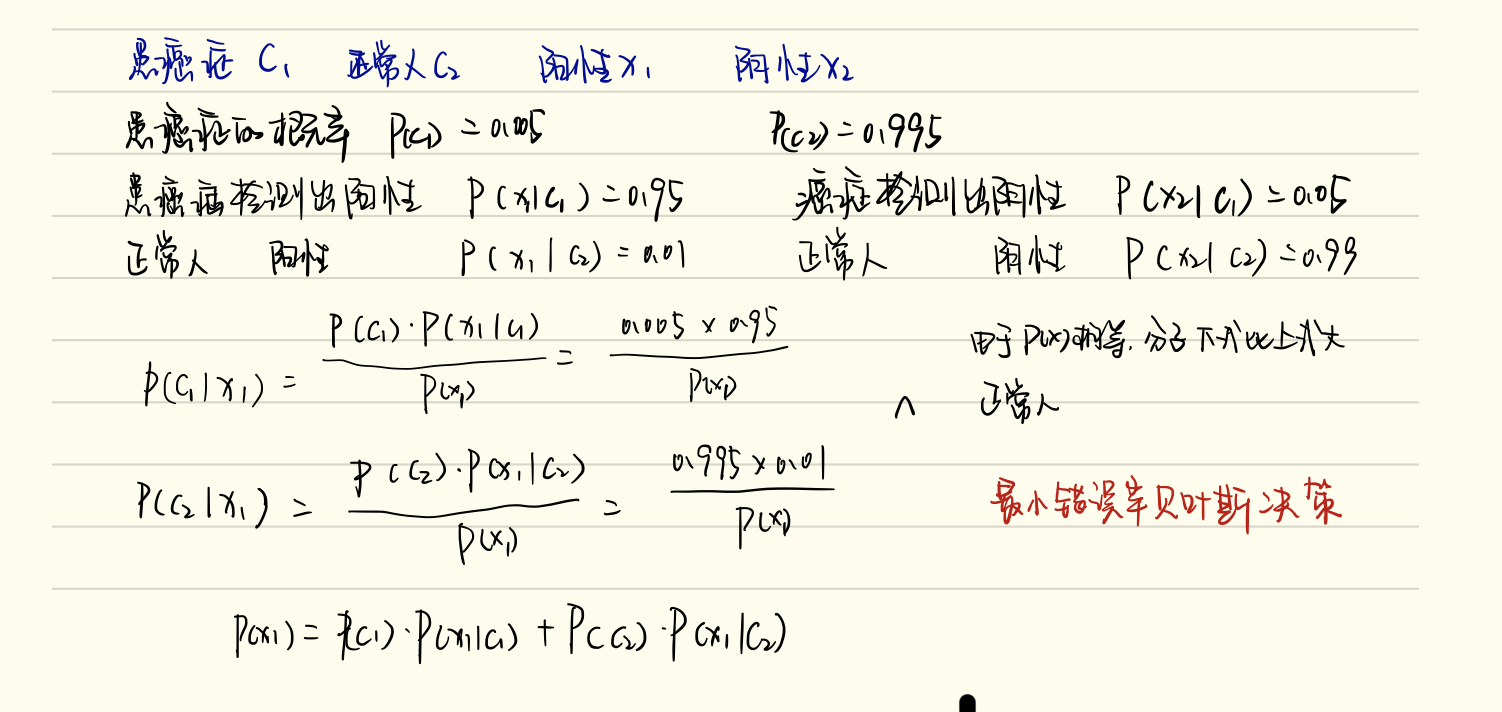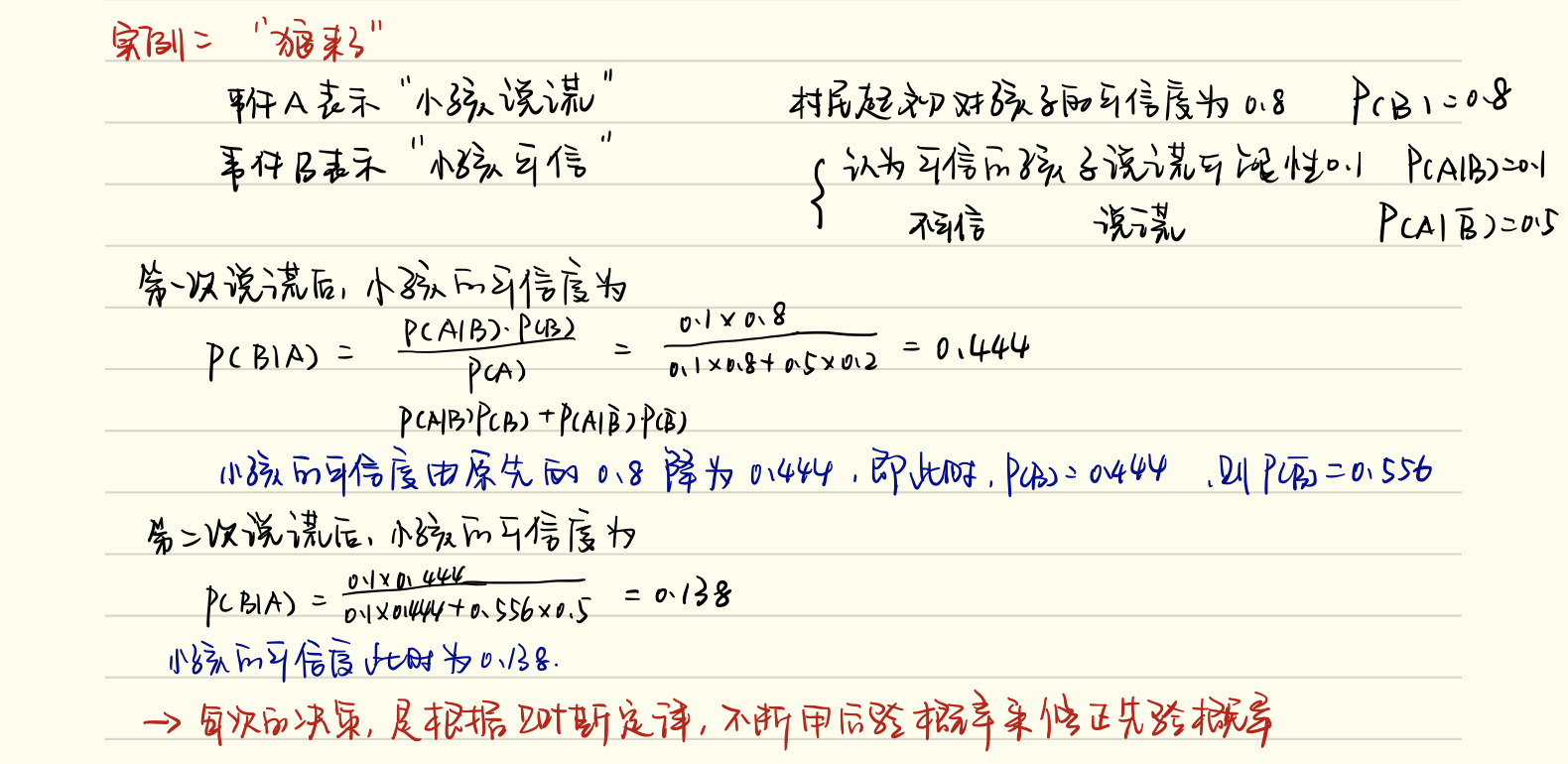• 本实训项目的主要内容是基于 Python 语言搭建朴素贝叶斯分类器，并使用sklearn 实现新闻文本进行主题分类的功能。 朴素贝叶斯分类算法流程 import numpy as np class NaiveBayesClassifier(ob
简介 朴素贝叶斯分类算法是基于贝叶斯理论和特征条件独立假设的分类算法。对于给定的训练集，首先基于特征条件独立假设学习数据的概率分布。然后基于此模型，对于给定的特征数据x，利用贝叶斯定理计算出标签y。朴素贝叶斯分类算法实现简单，预测效率很高，是一种常用的分类算法。 本实训项目的主要内容是基于 Python 语言搭建朴素贝叶斯分类器，并使用sklearn 实现新闻文本进行主题分类的功能。
朴素贝叶斯分类算法流程
import numpy as np

class NaiveBayesClassifier(object):
def __init__(self):
'''
self.label_prob表示每种类别在数据中出现的概率
例如，{0:0.333, 1:0.667}表示数据中类别0出现的概率为0.333，类别1的概率为0.667
'''
self.label_prob = {}
'''
self.condition_prob表示每种类别确定的条件下各个特征出现的概率
例如训练数据集中的特征为 [[2, 1, 1],
[1, 2, 2],
[2, 2, 2],
[2, 1, 2],
[1, 2, 3]]
标签为[1, 0, 1, 0, 1]
那么当标签为0时第0列的值为1的概率为0.5，值为2的概率为0.5;
当标签为0时第1列的值为1的概率为0.5，值为2的概率为0.5;
当标签为0时第2列的值为1的概率为0，值为2的概率为1，值为3的概率为0;
当标签为1时第0列的值为1的概率为0.333，值为2的概率为0.666;
当标签为1时第1列的值为1的概率为0.333，值为2的概率为0.666;
当标签为1时第2列的值为1的概率为0.333，值为2的概率为0.333,值为3的概率为0.333;
因此self.label_prob的值如下：
{
0:{
0:{
1:0.5
2:0.5
}
1:{
1:0.5
2:0.5
}
2:{
1:0
2:1
3:0
}
}
1:
{
0:{
1:0.333
2:0.666
}
1:{
1:0.333
2:0.666
}
2:{
1:0.333
2:0.333
3:0.333
}
}
}
'''
self.condition_prob = {}
def fit(self, feature, label):
'''
对模型进行训练，需要将各种概率分别保存在self.label_prob和self.condition_prob中
:param feature: 训练数据集所有特征组成的ndarray
:param label:训练数据集中所有标签组成的ndarray
:return: 无返回
'''

#********* Begin *********#
row_num = len(feature)
col_num = len(feature)
for c in label:
if c in self.label_prob:
self.label_prob[c] += 1
else:
self.label_prob[c] = 1

for key in self.label_prob.keys():
# 计算每种类别在数据集中出现的概率
self.label_prob[key] /= row_num
# 构建self.condition_prob中的key
self.condition_prob[key] = {}
for i in range(col_num):
self.condition_prob[key][i] = {}
for k in np.unique(feature[:, i], axis=0):
self.condition_prob[key][i][k] = 0

for i in range(len(feature)):
for j in range(len(feature[i])):
if feature[i][j] in self.condition_prob[label[i]]:
self.condition_prob[label[i]][j][feature[i][j]] += 1
else:
self.condition_prob[label[i]][j][feature[i][j]] = 1

for label_key in self.condition_prob.keys():
for k in self.condition_prob[label_key].keys():
total = 0
for v in self.condition_prob[label_key][k].values():
total += v
for kk in self.condition_prob[label_key][k].keys():
#计算每种类别确定的条件下各个特征出现的概率
self.condition_prob[label_key][k][kk] /= total
#********* End *********#

def predict(self, feature):
'''
对数据进行预测，返回预测结果
:param feature:测试数据集所有特征组成的ndarray
:return:
'''
# ********* Begin *********#
result = []
#对每条测试数据都进行预测
for i, f in enumerate(feature):
#可能的类别的概率
prob = np.zeros(len(self.label_prob.keys()))
ii = 0
for label, label_prob in self.label_prob.items():
#计算概率
prob[ii] = label_prob
for j in range(len(feature)):
prob[ii] *= self.condition_prob[label][j][f[j]]
ii += 1
#取概率最大的类别作为结果
result.append(list(self.label_prob.keys())[np.argmax(prob)])
return np.array(result)
#********* End *********#


拉普拉斯平滑
import numpy as np

class NaiveBayesClassifier(object):
def __init__(self):
'''
self.label_prob表示每种类别在数据中出现的概率
例如，{0:0.333, 1:0.667}表示数据中类别0出现的概率为0.333，类别1的概率为0.667
'''
self.label_prob = {}
'''
self.condition_prob表示每种类别确定的条件下各个特征出现的概率
例如训练数据集中的特征为 [[2, 1, 1],
[1, 2, 2],
[2, 2, 2],
[2, 1, 2],
[1, 2, 3]]
标签为[1, 0, 1, 0, 1]
那么当标签为0时第0列的值为1的概率为0.5，值为2的概率为0.5;
当标签为0时第1列的值为1的概率为0.5，值为2的概率为0.5;
当标签为0时第2列的值为1的概率为0，值为2的概率为1，值为3的概率为0;
当标签为1时第0列的值为1的概率为0.333，值为2的概率为0.666;
当标签为1时第1列的值为1的概率为0.333，值为2的概率为0.666;
当标签为1时第2列的值为1的概率为0.333，值为2的概率为0.333,值为3的概率为0.333;
因此self.label_prob的值如下：
{
0:{
0:{
1:0.5
2:0.5
}
1:{
1:0.5
2:0.5
}
2:{
1:0
2:1
3:0
}
}
1:
{
0:{
1:0.333
2:0.666
}
1:{
1:0.333
2:0.666
}
2:{
1:0.333
2:0.333
3:0.333
}
}
}
'''
self.condition_prob = {}

def fit(self, feature, label):
'''
对模型进行训练，需要将各种概率分别保存在self.label_prob和self.condition_prob中
:param feature: 训练数据集所有特征组成的ndarray
:param label:训练数据集中所有标签组成的ndarray
:return: 无返回
'''

#********* Begin *********#
row_num = len(feature)
col_num = len(feature)
unique_label_count = len(set(label))

for c in label:
if c in self.label_prob:
self.label_prob[c] += 1
else:
self.label_prob[c] = 1

for key in self.label_prob.keys():
# 计算每种类别在数据集中出现的概率，拉普拉斯平滑
self.label_prob[key] += 1
self.label_prob[key] /= (unique_label_count+row_num)

# 构建self.condition_prob中的key
self.condition_prob[key] = {}
for i in range(col_num):
self.condition_prob[key][i] = {}
for k in np.unique(feature[:, i], axis=0):
self.condition_prob[key][i][k] = 1

for i in range(len(feature)):
for j in range(len(feature[i])):
if feature[i][j] in self.condition_prob[label[i]]:
self.condition_prob[label[i]][j][feature[i][j]] += 1

for label_key in self.condition_prob.keys():
for k in self.condition_prob[label_key].keys():
#拉普拉斯平滑
total = len(self.condition_prob[label_key].keys())
for v in self.condition_prob[label_key][k].values():
total += v
for kk in self.condition_prob[label_key][k].keys():
# 计算每种类别确定的条件下各个特征出现的概率
self.condition_prob[label_key][k][kk] /= total
#********* End *********#

def predict(self, feature):
'''
对数据进行预测，返回预测结果
:param feature:测试数据集所有特征组成的ndarray
:return:
'''

result = []
# 对每条测试数据都进行预测
for i, f in enumerate(feature):
# 可能的类别的概率
prob = np.zeros(len(self.label_prob.keys()))
ii = 0
for label, label_prob in self.label_prob.items():
# 计算概率
prob[ii] = label_prob
for j in range(len(feature)):
prob[ii] *= self.condition_prob[label][j][f[j]]
ii += 1
# 取概率最大的类别作为结果
result.append(list(self.label_prob.keys())[np.argmax(prob)])
return np.array(result)


新闻文本主题分类
from sklearn.feature_extraction.text import CountVectorizer  # 从sklearn.feature_extraction.text里导入文本特征向量化模块
from sklearn.naive_bayes import MultinomialNB
from sklearn.feature_extraction.text import TfidfTransformer

def news_predict(train_sample, train_label, test_sample):
'''
训练模型并进行预测，返回预测结果
:param train_sample:原始训练集中的新闻文本，类型为ndarray
:param train_label:训练集中新闻文本对应的主题标签，类型为ndarray
:test_sample:原始测试集中的新闻文本，类型为ndarray
'''

# ********* Begin *********#
vec = CountVectorizer()
train_sample = vec.fit_transform(train_sample)
test_sample = vec.transform(test_sample)

tfidf = TfidfTransformer()

train_sample = tfidf.fit_transform(train_sample)
test_sample = tfidf.transform(test_sample)

mnb = MultinomialNB(alpha=0.01)  # 使用默认配置初始化朴素贝叶斯
mnb.fit(train_sample, train_label)  # 利用训练数据对模型参数进行估计
predict = mnb.predict(test_sample)  # 对参数进行预测
return predict
# ********* End *********#

感谢大家的支持！！！！！记得点赞！！！
展开全文机器学习
• 朴素贝叶斯分类器假设每个属性相互独立，. 对于所有类别来说，相同，因此朴素贝叶斯分类器对的类标判别.  令表示训练集中第类样本组成的集合，表示类别数，则类先验概率（拉普拉斯平滑） 对于离散属性，令表示...
理论
记为类标，为输入，由贝叶斯公式，. 朴素贝叶斯分类器假设每个属性相互独立，. 对于所有类别来说，相同，因此朴素贝叶斯分类器对的类标判别.
令表示训练集中第类样本组成的集合，表示类别数，则类先验概率（拉普拉斯平滑）

对于离散属性，令表示类标为、属性取值为的样本组成的集合，为第个属性的可能取值数，则类先验概率（拉普拉斯平滑）

对于连续属性，假设服从正态分布，即，用概率密度表示

代码
# -*- coding: utf-8 -*-
"""
Created on Mon Aug 27 21:44:30 2018

Naive Bayesian Classifier
From 'Machine Learning, Zhihua Zhou' Ch7
Model: P166 problem 7.3
With Laplacian Smoothing
Dataset: P84 watermelon_3.0 (watermelon_3.0.npy)

@author: weiyx15
"""

import numpy as np

class Naive_Bayesian_Classifier:
def Gausian_density(self, x, mu, sigma):
return 1/np.sqrt(2*np.pi)/sigma*np.exp(-(x-mu)*(x-mu)/2/sigma/sigma)

self.d1 = dic['arr_0']  # number of discrete property
self.d2 = dic['arr_1']  # number of continuous property
self.k = dic['arr_2']   # number of categories
self.xs = dic['arr_3']  # discrete property range
self.x = dic['arr_4']   # training data
self.y = dic['arr_5']   # training label
self.m = self.x.shape# training data size

def __init__(self):

''' pxy =
p(x0=0|c0), p(x0=1|c0), ..., p(x0=x0m|c0)
p(x0=0|c1), p(x0=1|c1), ..., p(x0=x0m|c1)
...
p(x0=0|ck), p(x0=1|ck), ..., p(x0=x0m|ck)

p(x1=0|c0), p(x1=1|c0), ..., p(x1=x1m|c0)
p(x1=0|c1), p(x1=1|c1), ..., p(x1=x1m|c1)
...
p(x1=0|ck), p(x1=1|ck), ..., p(x1=x1m|ck)

...
...
...
'''
def train(self):
# deal with discrete property
self.py = np.ones((self.k,))
for i in range(self.m):
self.py[self.y[i]] = self.py[self.y[i]] + 1
pxylen = self.d1 * self.k
self.pxy = [list()] * pxylen
for i in range(self.d1*self.k):
self.pxy[i] =  * self.xs[int(i/self.k)]
for i in range(self.m):
for j in range(self.d1):
self.pxy[self.y[i]*self.d1 + j][int(self.x[i,j])] =\
self.pxy[self.y[i]*self.d1 + j][int(self.x[i,j])] + 1
for i in range(self.d1*self.k):
for j in range(len(self.pxy[i])):
self.pxy[i][j] = self.pxy[i][j] \
/ (self.py[i%int(self.k)] + self.xs[int(i/self.k)] - 1)
self.py = self.py / (self.m + self.k)
# deal with continuous property
self.mu = np.zeros((self.k, self.d2))
self.sigma = np.zeros((self.k, self.d2))
for i in range(self.k):
for j in range(self.d2):
ij_ind = np.where(self.x[:, self.d1+j])
ij_sample = self.x[ij_ind, self.d1+j]
ij_sample = ij_sample.reshape((ij_sample.size,))
self.mu[i][j] = np.mean(ij_sample)
self.sigma[i][j] = np.std(ij_sample, ddof=1)

def test(self, xt):
pmax = -np.inf
idmax = -1
for i in range(self.k):
pi = np.log2(self.py[i])
for j in range(self.d1):
pi = pi + np.log2(self.pxy[self.d1*i+j][int(xt[j])])
for j in range(self.d2):
pi = pi + np.log2(self.Gausian_density\
(xt[self.d1+j], self.mu[i][j], self.sigma[i][j]))
if pi > pmax:
pmax = pi
idmax = i
return idmax

if __name__ == '__main__':
nbc = Naive_Bayesian_Classifier()
nbc.train()
ans = nbc.test([1, 0, 0, 0, 0, 0, 0.697, 0.46])
# test data: 'Machine Learning, Zhihua Zhou' P151

展开全文西瓜书 机器学习
• 通过两个实例理解贝叶斯的思想与流程，然后梳理了朴素贝叶斯分类器的算法流程，最后从零开始实现了朴素分类器的算法。 文章目录1.起源、提出与贝叶斯公式2.以实例感受贝叶斯决策：癌症病人计算 问题3.以实例感受...
本文从贝叶斯与频率概率的对比入手理解贝叶斯决策的思维方式。通过两个实例理解贝叶斯的思想与流程，然后梳理了朴素贝叶斯分类器的算法流程，最后从零开始实现了朴素分类器的算法。

文章目录
1.起源、提出与贝叶斯公式2.以实例感受贝叶斯决策：癌症病人计算 问题3.以实例感受贝叶斯修正先验概率：狼来了4.朴素贝叶斯分类器5.代码实现1.数据集载入，划分训练集与测试集2.计算先验概率3.计算类条件概率4.先验概率*类条件概率
6. 参考文献

1.起源、提出与贝叶斯公式
贝叶斯派别主要是与古典统计学相比较区分的。
古典统计学基于大数定理，将一件事件经过大量重复实验得到的频率作为事件发生的概率，如常说的掷一枚硬币，正面朝上的概率为0.5。但，如果事先知道硬币的密度分布并不均匀，那此时的概率是不是就不为0.5呢？这种不同于“非黑即白”的思考方式，就是贝叶斯式的思考方式。
贝叶斯除了提出上述思考方式之外，还特别提出了举世闻名的贝叶斯定理。贝叶斯公式：

P

(

B

i

∣

A

)

=

P

(

B

i

)

P

(

A

∣

B

i

)

∑

j

=

1

n

P

(

B

j

)

P

(

A

∣

B

j

)

P(B_i|A) = \frac{P(B_i)P(A|B_i)}{\sum_{j=1}^nP(B_j)P(A|B_j)}

这里通过全概率公式，我们可以将上式等同于

P

(

B

∣

A

)

=

P

(

B

)

P

(

A

∣

B

)

P

(

A

)

=

P

(

B

)

P

(

A

∣

B

)

P

(

A

)

P(B|A) = \frac{P(B)P(A|B)}{P(A)} = P(B)\frac{P(A|B)}{P(A)}

右边的分式中，分子的P(A)称为先验概率，是B事件未发生前，对A事件概率的判断；P(A|B)即是在B事件发生之后，对A事件发生的后验概率。这整个分式我们也称之为‘’可能性函数(Likelyhood)‘’，这是一个调整因子，对A事件未发生之前B的概率进行调整，以得到A事件发生的前提下B事件发生的后验概率P(B|A)。
以一句话与一张图概括：贝叶斯定理是一种在已知其他概率的情况下求概率的方法。2.以实例感受贝叶斯决策：癌症病人计算 问题

有一家医院为了研究癌症的诊断，对一大批人作了一次普查，给每人打了试验针，然后进行统计，得到如下统计数字：
这批人中，每1000人有5个癌症病人；这批人中，每100个正常人有1人对试验的反应为阳性这批人中，每100个癌症病人有95入对试验的反应为阳性。
通过普查统计，该医院可开展癌症诊断。现在某人试验结果为阳性，诊断结果是什么?(全是公式实在不好打，就用自己笔记代替啦)
上述例子是基于最小错误率贝叶斯决策，即哪类的概率大判断为哪类，也是我们常见的分类决策方法。但是将正常人错判为癌症病人，与将癌症病人错判为正常人的损失是不一样的。将正常人错判为癌症病人，会给他带来短期的精神负担，造成一定的损失，这个损失比较小。如果把癌症病人错判为正常人，致使患者失去挽救的机会，这个损失就大了。这两种不同的错判所造成损失的程度是有显著差别的。
所以，在决策时还要考虑到各种错判所造成的不同损失，由此提出了最小风险贝叶斯决策。
我们将

I

i

j

I_{ij}

记为将j类误判为i类所造成的损失。此处类别为2，如将样本x判为癌症病人

c

1

c_1

造成损失的数学期望为：

R

1

=

I

11

P

(

C

1

∣

X

)

+

I

12

P

(

C

2

∣

X

)

​

R_1=I_{11}P(C_1|X)+I_{12}P(C_2|X)​

同理，将样本x判为癌症病人

c

2

c_2

造成损失的数学期望为

R

2

=

I

21

P

(

C

1

∣

X

)

+

I

22

P

(

C

2

∣

X

)

R_2=I_{21}P(C_1|X)+I_{22}P(C_2|X)

选择最小风险作为决策准则，若

R

1

&lt;

R

2

R_1&lt;R_2

，则样本X

ϵ

R

1

\epsilon R_1

，否则X

ϵ

R

2

\epsilon R_2

3.以实例感受贝叶斯修正先验概率：狼来了

给出一些合理解释：
事件A表示:“小孩说谎”；事件B表示:“小孩可信”。
村民起初对这个小孩的信任度为0.8,即P(B)=0.8。
我们认为可信的孩子说谎的可能性为0.1。即P(A|B)=0.1。
不可信的孩子说谎的可能性为0.5,即P(A|^B)=0.5(用 ^B表示B的对立事件)。
求小孩第一次说谎、第二次说谎后，村民对这个小孩的信任度从P(B)=0.8会发生什么变化?由此我们可以得到贝叶斯决策另一个道理，**贝叶斯决策会不断用后验概率，逐渐修正先验概率。**投射到现实生活，我们也可以理解为当对某件事进行判断决策时，关于此事得到的信息越多，对此事的决策越准，而绝非yes/no的五五开决策。

还是狼来的例子，如果这个孩子要改邪归正，他需要多少次才能把信任度提高到80%？

如果要把信任度提高，那接下来就需要通过几次 不说谎 的经历来修正村民的观念，那可信度的计算，要记得将P(A|B)换成P((fei)A|B)，(A上方的横线打不出来…)，就是可信的孩子不说谎的可能性为1-P(A|B)=1-0.1，同样，不可信的孩子不说谎的概率为1-0.5
此时，我们就要用代码来实现而不是手算啦。代码如下：
def calculateTrustDegree(pb):
PB_A = float((1-PA_B)*pb)/((1-PA_B)*pb+(1-PA_Bf)*(1-pb))
return PB_A
PA_B = 0.1
PA_Bf = 0.5
pb = 0.138
N = 0
while(pb <=0.8):
pb = calculateTrustDegree(pb)
N+=1
print("He need {0} times with honest saying,villager can trust him again.".format(N))

# N = 6
# He need 6 times with honest saying,villager can trust him again.

4.朴素贝叶斯分类器
贝叶斯分类算法是一大类分类算法的总称。
贝叶斯分类算法以样本可能属于某类的概率来作为分类依据。
朴素贝叶斯分类算法是贝叶斯分类算法中最简单的一种，采用了**“属性条件独立性假设”**：对已知类别，假设所有属性互相独立，也就是在概率的计算时，可以将一个类别的每个属性直接相乘。这在概率论中应该学过，两个事件独立时，两个事件同时发生的概率等于两个事件分别发生的乘积。
给定一个属性值，其属于某个类的概率叫做条件概率。对于一个给定的类值，将每个属性的条件概率相乘，便得到一个数据样本属于某个类的概率。
我们可以通过计算样本归属于每个类的概率，然后选择具有最高概率的类来做预测。
我们以鸢尾花分类实例来过算法流程，并不适用sklearn库自实现朴素贝叶斯分类器。

实例数据集介绍：鸢尾花数据集包含4维的特征，共三类150个样本，每类均有50个样本。

算法流程概括如下：
计算样本属于某类的先验概率，属于A类的概率为

属

于

A

类

的

样

本

数

所

有

样

本

数

\frac{属于A类的样本数}{所有样本数}

，以此类推计算类条件概率，离散值通过类别数量比值，此数据集的属性特征为连续值所以通过 概率密度函数 来计算。首先计算在一个属性的前提下，该样本属于某类的概率；相乘合并所有属性的概率，即为某个数据样本属于某类的类条件概率
计算每个特征属于每类的条件概率
概率密度函数实现计算每个属性的均值和方差按类别提取属性特征，这里会得到 类别数目*属性数目 组 （均值，方差） 按类别将每个属性的条件概率相乘，如下所示
判断为A类的概率：p(A|特征1)*p(A|特征2)*p(A|特征3)*p(A|特征4)…判断为B类的概率：p(B|特征1)*p(B|特征2)*p(B|特征3)*p(B|特征4)…判断为C类的概率：p(C|特征1)*p(C|特征2)*p(C|特征3)*p(C|特征4)…  先验概率*类条件概率，回顾一下贝叶斯公式，

P

(

B

i

∣

A

)

=

P

(

B

i

)

P

(

A

∣

B

i

)

∑

j

=

1

n

P

(

B

j

)

P

(

A

∣

B

j

)

P(B_i|A) = \frac{P(B_i)P(A|B_i)}{\sum_{j=1}^nP(B_j)P(A|B_j)}

。由于样本确定时，贝叶斯公式的分母都是相同的。所以判断样本属于哪类只需要比较分子部分：先验概率*类条件概率，最终属于哪类的概率最大，则判别为哪类，此处为最小错误率贝叶斯分类，若采用最小风险需要加上判断为每个类别的风险损失值。
5.代码实现
1.数据集载入，划分训练集与测试集
data_df = pd.read_csv('IrisData.csv')
def splitData(data_list,ratio):
train_size = int(len(data_list)*ratio)
random.shuffle(data_list)
train_set = data_list[:train_size]
test_set = data_list[train_size:]
return train_set,test_set

data_list = np.array(data_df).tolist()
trainset,testset = splitData(data_list,ratio = 0.7)
print('Split {0} samples into {1} train and {2} test samples '.format(len(data_df), len(trainset), len(testset)))

# Split 150 samples into 105 train and 45 test samples

2.计算先验概率
此时需要先知道数据集中属于各类别的样本分别有多少。我们通过一个函数实现按类别划分数据。
两个返回值分别为划分好的数据字典，以及划分好的数据集中每个类别的样本数
def seprateByClass(dataset):
seprate_dict = {}
info_dict = {}
for vector in dataset:
if vector[-1] not in seprate_dict:
seprate_dict[vector[-1]] = []
info_dict[vector[-1]] = 0
seprate_dict[vector[-1]].append(vector)
info_dict[vector[-1]] +=1
return seprate_dict,info_dict

train_separated,train_info = seprateByClass(trainset)

# train_info：
# {'Setosa': 41, 'Versicolour': 33, 'Virginica': 31}

计算属于每个类别的先验概率
def calulateClassPriorProb(dataset,dataset_info):
dataset_prior_prob = {}
sample_sum = len(dataset)
for class_value, sample_nums in dataset_info.items():
dataset_prior_prob[class_value] = sample_nums/float(sample_sum)
return dataset_prior_prob

prior_prob = calulateClassPriorProb(trainset,train_info)

#{'Setosa': 0.3904761904761905,
# 'Versicolour': 0.3142857142857143,
# 'Virginica': 0.29523809523809524}

3.计算类条件概率
3.1 首先计算每个特征属于每类的条件概率，前面说过这里我们使用概率密度函数来计算
概率密度函数实现：
方差公式：$var = \frac{\sum(x-avg)^{2}}{n-1} ， 概 率 密 度 函 数 ： ，概率密度函数： p(xi|c) = \frac{1}{\sqrt{2\pi}\sigma_{c,i}}exp(-\frac{(xi-mean_{c,i}){2}}{2\sigma_{c,i}{2}})$ ,

σ

\sigma

是标准差（方差开方）
# 均值
def mean(list):
list = [float(x) for x in list] #字符串转数字
return sum(list)/float(len(list))
# 方差
def var(list):
list = [float(x) for x in list]
avg = mean(list)
var = sum([math.pow((x-avg),2) for x in list])/float(len(list)-1)
return var
# 概率密度函数
def calculateProb(x,mean,var):
exponent = math.exp(math.pow((x-mean),2)/(-2*var))
p = (1/math.sqrt(2*math.pi*var))*exponent
return p

每个属性特征属于每类的条件概率是个组合。举例来说，这里有3个类和4个数值属性，然后我们需要每一个属性（4）和类（3）的组合的类条件概率。
为了得到这12个概率密度函数，那我们需要提前知道这12个属性分别的均值和方差，才可以带入到上述概率密度函数中计算。
计算每个属性的均值和方差：
def summarizeAttribute(dataset):
dataset = np.delete(dataset,-1,axis = 1) # delete label
summaries = [(mean(attr),var(attr)) for attr in zip(*dataset)]
return summaries

summary = summarizeAttribute(trainset)
#[(5.758095238095239, 0.7345732600732595),
# (3.065714285714285, 0.18592857142857133),
# (3.5533333333333323, 3.2627051282051274),
# (1.1142857142857148, 0.6014285714285714)]

按类别提取属性特征，这里会得到 类别数目*属性数目 组 （均值，方差）
def summarizeByClass(dataset):
dataset_separated,dataset_info = seprateByClass(dataset)
summarize_by_class = {}
for classValue, vector in dataset_separated.items():
summarize_by_class[classValue] = summarizeAttribute(vector)
return summarize_by_class

train_Summary_by_class = summarizeByClass(trainset)
#{'Setosa': [(4.982926829268291, 0.12445121951219511),
#  (3.3975609756097565, 0.1417439024390244),
#  (1.4707317073170731, 0.03412195121951221),
#  (0.24390243902439032, 0.012024390243902434)],
# 'Versicolour': [(5.933333333333334, 0.2766666666666667),
#  (2.7909090909090906, 0.08960227272727272),
#  (4.254545454545454, 0.23755681818181815),
#  (1.33030303030303, 0.03905303030303031)],
# 'Virginica': [(6.596774193548387, 0.5036559139784946),
#  (2.9193548387096775, 0.10427956989247314),
#  (5.5612903225806445, 0.37711827956989247),
#  (2.0354838709677416, 0.06369892473118278)]}


按类别将每个属性的条件概率相乘。
我们前面已经将训练数据集按类别分好，这里就可以实现，输入的测试数据依据每类的每个属性（就那个类别数*属性数的字典）计算属于某类的类条件概率。
def calculateClassProb(input_data,train_Summary_by_class):
prob = {}
for class_value, summary in train_Summary_by_class.items():
prob[class_value] = 1
for i in range(len(summary)):
mean,var = summary[i]
x = input_data[i]
p = calculateProb(x,mean,var)
prob[class_value] *=p
return prob

input_vector = testset
input_data = input_vector[:-1]
train_Summary_by_class = summarizeByClass(trainset)
class_prob = calculateClassProb(input_data,train_Summary_by_class)

#{'Setosa': 3.3579279836005993,
# 'Versicolour': 1.5896628317396685e-07,
# 'Virginica': 5.176617264913899e-12}

4.先验概率*类条件概率
朴素贝叶斯分类器
def bayesianPredictOneSample(input_data):
prior_prob = calulateClassPriorProb(trainset,train_info)
train_Summary_by_class = summarizeByClass(trainset)
classprob_dict = calculateClassProb(input_data,train_Summary_by_class)
result = {}
for class_value,class_prob in classprob_dict.items():
p = class_prob*prior_prob[class_value]
result[class_value] = p
return max(result,key=result.get)

终于把分类器写完啦，接下来就让我们看看测试数据的结果！
单个样本测试：
input_vector = testset
input_data = input_vector[:-1]
result = bayesianPredictOneSample(input_data)
print("the sameple is predicted to class: {0}.".format(result))

# the sameple is predicted to class: Versicolour.

看看分类准确率
def calculateAccByBeyesian(dataset):
correct = 0
for vector in dataset:
input_data = vector[:-1]
label = vector[-1]
result = bayesianPredictOneSample(input_data)
if result == label:
correct+=1
return correct/len(dataset)

acc = calculateAccByBeyesian(testset)
# 0.9333333333333333

全部代码及数据集已上传至github，也可访问我的博客，第一次写博客不足之处欢迎大家提出交流学习。最近会持续性进行输出的~而且每一次输出都是一次新的输入。
6. 参考文献
机器学习之用Python从零实现贝叶斯分类器
知乎-你对贝叶斯统计都有怎样的理解？
贝叶斯公式由浅入深大讲解—AI基础算法入门
先验乘后验贝叶斯定理
展开全文机器学习
• 主要为大家详细介绍了python实现朴素贝叶斯分类器，具有一定的参考价值，感兴趣的小伙伴们可以参考一下
• python写的简单的朴素贝叶斯分类器，一共有两个主文件，nbayes1和nbayes1_run1还有两个训练数据文件，望大家多多指教。
• 主要介绍了Python实现的朴素贝叶斯分类器,结合具体实例形式分析了基于Python实现的朴素贝叶斯分类器相关定义与使用技巧,需要的朋友可以参考下
• ... 0. 前言 贝叶斯分类是一类分类算法的总称...本文由本人学习贝叶斯分类器过程中的笔记，再加上使用Python进行文本分类实战组成。 1. 贝叶斯决策论（Bayesian decision theory） 贝叶斯决策论是概率框架下实施决策...

Github: github.com/yingzk/MyML  博 客: www.yingjoy.cn/
0. 前言
贝叶斯分类是一类分类算法的总称，这类算法均以贝叶斯定理为基础，故统称为贝叶斯分类。本文由本人学习贝叶斯分类器过程中的笔记，再加上使用Python进行文本分类实战组成。
1. 贝叶斯决策论（Bayesian decision theory）
贝叶斯决策论是概率框架下实施决策的基本方法。对于分类任务来说，在所有相关概率都已知的情况下，贝叶斯决策论考虑如何基于这些概率和误判损失来选择最优的类别标记。
假设由N种可能的类别标记，,是将一个真实标记为的样本误差分类为所产生的损失，基于后验概率可获得将样本分类为所产生的期望损失(expected loss)，即在样本上的“条件风险”(conditional risk)主要任务是寻找一个判定准则以最小化总体风险显然，对每个样本,若能最小化条件风险,则总体风险也将被最小化
由此产生了贝叶斯准则(Bayes decision rule):
为最小化总体风险，只需在每个样本上选择那个能使条件风险最小的类别标记此时，称为贝叶斯最优分类器（Bayse optimal classifier），与之对应的总体风险称为贝叶斯风险（Bayes risk）.反映了分类器所能达到的最好性能。
若目标是最小化分类错误率，则误判损失可写为此时风险条件为：最小化分类错误率的贝叶斯最优分类器为基于贝叶斯定理，关于这个公式的证明，很容易，依照条件概率的定义即可得到，感兴趣的读者可以百度一下。这个定理虽然很简单，但是却建立起先验概率和后验概率相互转化的桥梁。为类先验概率称为类条件概率或者似然

先验概率： 根据以往经验和分析得到的概率。后验概率：后验概率是基于新的信息，修正原来的先验概率后所获得的更接近实际情况的概率估计。

对于类先验概率就是样本空间中各类样本所占的比例，根据大数定理（当样本足够多时，频率趋于稳定等于其概率），这样当训练样本充足时，可以使用各类出现的频率来代替。因此只剩下类条件概率,它表达的意思是在类别c中出现x的概率，它涉及到属性的联合概率问题，若只有一个离散属性还好，当属性多时采用频率估计起来就十分困难，因此这里一般采用极大似然法进行估计。
###2. 极大似然估计
极大似然估计（Maximum Likelihood Estimation，简称MLE），是一种根据数据采样来估计概率分布的经典方法。常用的策略是先假定总体具有某种确定的概率分布，再基于训练样本对概率分布的参数进行估计。运用到类条件概率中，假设服从一个参数为θ的分布，问题就变为根据已知的训练样本来估计。极大似然法的核心思想就是：估计出的参数使得已知样本出现的概率最大，即使得训练数据的似然最大。
令表示训练集中第类样本组成的集合，假设这些样本是独立同分布的，则参数对数据集的似然是对进行极大似然估计，就是去寻找能最大似然的参数值. 直观上看，极大似然估计是视图在所有可能取值中，找到一个能使数据出现的“可能性”最大的值
连乘操作使得求解变得复杂（容易造成下溢），一般我们使用对数似然(log-likelihood)此时参数的极大似然估计为所以，贝叶斯分类器的训练过程就是参数估计。总结最大似然法估计参数的过程，一般分为以下四个步骤：

1.写出似然函数；2.对似然函数取对数，并整理；3.求导数，令偏导数为0，得到似然方程组；4.解似然方程组，得到所有参数即为所求。

3. 朴素贝叶斯分类器
朴素贝叶斯分类是一种十分简单的分类算法，叫它朴素贝叶斯分类是因为这种方法的思想真的很朴素，朴素贝叶斯的思想基础是这样的：对于给出的待分类项，求解在此项出现的条件下各个类别出现的概率，哪个最大，就认为此待分类项属于哪个类别。通俗来说，就好比这么个道理，你在街上看到一个黑人，我问你你猜这哥们哪里来的，你十有八九猜非洲。为什么呢？因为黑人中非洲人的比率最高，当然人家也可能是美洲人或亚洲人，但在没有其它可用信息下，我们会选择条件概率最大的类别，这就是朴素贝叶斯的思想基础。
基于属性条件独立性假设，可以得到为属性数目,为在第个属性上的取值这就是朴素贝叶斯的表达式
这样，为每个样本估计类条件概率变成为每个样本的每个属性估计类条件概率。
对于离散属性 ，属性的条件概率可以估计为：对于连续属性， 若假设属性服从正态分布，则条件概率可以估计为：实例
这里用西瓜数据集3.0为例
--《周志华-西瓜书》
编号,色泽,根蒂,敲声,纹理,脐部,触感,密度,含糖率,好瓜
1,青绿,蜷缩,浊响,清晰,凹陷,硬滑,0.697,0.46,是
2,乌黑,蜷缩,沉闷,清晰,凹陷,硬滑,0.774,0.376,是
3,乌黑,蜷缩,浊响,清晰,凹陷,硬滑,0.634,0.264,是
4,青绿,蜷缩,沉闷,清晰,凹陷,硬滑,0.608,0.318,是
5,浅白,蜷缩,浊响,清晰,凹陷,硬滑,0.556,0.215,是
6,青绿,稍蜷,浊响,清晰,稍凹,软粘,0.403,0.237,是
7,乌黑,稍蜷,浊响,稍糊,稍凹,软粘,0.481,0.149,是
8,乌黑,稍蜷,浊响,清晰,稍凹,硬滑,0.437,0.211,是
9,乌黑,稍蜷,沉闷,稍糊,稍凹,硬滑,0.666,0.091,否
10,青绿,硬挺,清脆,清晰,平坦,软粘,0.243,0.267,否
11,浅白,硬挺,清脆,模糊,平坦,硬滑,0.245,0.057,否
12,浅白,蜷缩,浊响,模糊,平坦,软粘,0.343,0.099,否
13,青绿,稍蜷,浊响,稍糊,凹陷,硬滑,0.639,0.161,否
14,浅白,稍蜷,沉闷,稍糊,凹陷,硬滑,0.657,0.198,否
15,乌黑,稍蜷,浊响,清晰,稍凹,软粘,0.36,0.37,否
16,浅白,蜷缩,浊响,模糊,平坦,硬滑,0.593,0.042,否
17,青绿,蜷缩,沉闷,稍糊,稍凹,硬滑,0.719,0.103,否
复制代码
用上面数据训练一个朴素贝叶斯分类器，对测试例 “测1”进行分类：
编号,色泽,根蒂,敲声,纹理,脐部,触感,密度,含糖率,好瓜
测1,青绿,蜷缩,浊响,清晰,凹陷,硬滑,0.697,0.46,是
复制代码

实战 - 使用Python进行文本分类
要从文本中获取特征，需要先拆分文本。具体如何做？这里的特征是来自文本的词条（token），一个词条是字符的任意组合。可以把词条想象为单词，也可以使用非单词词条，如URL、IP地址或者任意其他字符串。
以在线社区的留言板为例。为了不影响社区的发展，我们要屏蔽侮辱性的言论，所以要构建一个快速过滤器，如果某条留言使用了负面或者侮辱性的语言，那么就该留言标识为内容不当。过滤这类内容是一个很常见的需求。对此问题建立两个类别，侮辱性和非侮辱性，使用1和0分别表示。
数据：
"my","dog","has","flea","problems","help","please","null"
"maybe","not","take","him","to","dog","park","stupid","null"
"my","dalmation","is","so","cute","I","love","him","null"
"stop","posting","stupid","worthless","garbage","null"
"mr","licks","ate","my","steak","how","to","stop","him","null"
复制代码
Python实现：
# _*_ coding:utf-8 _*_
import numpy as np

"""
导入数据， 1代表脏话
@ return postingList: 数据集
@ return classVec: 分类向量
"""
postingList = [['my', 'dog', 'has', 'flea', 'problems', 'help', 'please'],
['maybe', 'not', 'take', 'him', 'to', 'dog', 'park', 'stupid'],
['my', 'dalmation', 'is', 'so', 'cute', 'I', 'love', 'him'],
['stop', 'posting', 'stupid', 'worthless', 'garbage'],
['mr', 'licks', 'ate', 'my', 'steak', 'how', 'to', 'stop', 'him'],
['quit', 'buying', 'worthless', 'dog', 'food', 'stupid']]
classVec = [0, 1, 0, 1, 0, 1]
return postingList, classVec

def createVocabList(dataSet):
"""
创建词库
@ param dataSet: 数据集
@ return vocabSet: 词库
"""
vocabSet = set([])
for document in dataSet:
# 求并集
vocabSet = vocabSet | set(document)
return list(vocabSet)

def setOfWords2Vec(vocabList, inputSet):
"""
文本词向量.词库中每个词当作一个特征，文本中就该词，该词特征就是1，没有就是0
@ param vocabList: 词表
@ param inputSet: 输入的数据集
@ return returnVec: 返回的向量
"""
returnVec =  * len(vocabList)
for word in inputSet:
if word in vocabList:
returnVec[vocabList.index(word)] = 1
else:
print("单词: %s 不在词库中!" % word)
return returnVec

def trainNB0(trainMatrix, trainCategory):
"""
训练
@ param trainMatrix: 训练集
@ param trainCategory: 分类
"""
numTrainDocs = len(trainMatrix)
numWords = len(trainMatrix)
pAbusive = sum(trainCategory) / float(numTrainDocs)
#防止某个类别计算出的概率为0，导致最后相乘都为0，所以初始词都赋值1，分母赋值为2.
p0Num = np.ones(numWords)
p1Num = np.ones(numWords)
p0Denom = 2
p1Denom = 2
for i in range(numTrainDocs):
if trainCategory[i] == 1:
p1Num += trainMatrix[i]
p1Denom += sum(trainMatrix[i])
else:
p0Num += trainMatrix[i]
p0Denom += sum(trainMatrix[i])
# 这里使用log函数，方便计算，因为最后是比较大小，所有对结果没有影响。
p1Vect = np.log(p1Num / p1Denom)
p0Vect = np.log(p0Num / p0Denom)
return p0Vect, p1Vect, pAbusive

def classifyNB(vec2Classify,p0Vec,p1Vec,pClass1):
"""
判断大小
"""
p1 = sum(vec2Classify*p1Vec)+np.log(pClass1)
p0 = sum(vec2Classify*p0Vec)+np.log(1-pClass1)
if p1>p0:
return 1
else:
return 0

def testingNB():
myVocabList = createVocabList(listOPosts)
trainMat=[]
for postinDoc in listOPosts:
trainMat.append(setOfWords2Vec(myVocabList, postinDoc))
p0V,p1V,pAb = trainNB0(np.array(trainMat),np.array(listClasses))
testEntry = ['love', 'my', 'dalmation']
thisDoc = np.array(setOfWords2Vec(myVocabList, testEntry))
print(testEntry,'classified as: ',classifyNB(thisDoc,p0V,p1V,pAb))
testEntry = ['stupid', 'garbage']
thisDoc = np.array(setOfWords2Vec(myVocabList, testEntry))
print(testEntry,'classified as: ',classifyNB(thisDoc,p0V,p1V,pAb))

if __name__=='__main__':
testingNB()
复制代码

展开全文• 贝叶斯分类器 贝叶斯分类器的分类原理是通过某对象的先验概率，利用贝叶斯公式计算出其后验概率，即该对象属于某一类的概率，选择具有最大后验概率的类作为该对象所属的类。 介绍一下先验概率：是指根据以往经验和...机器学习
• 主要介绍了Python实现朴素贝叶斯分类器的方法,详细分析了朴素贝叶斯分类器的概念、原理、定义、使用方法及相关操作注意事项,需要的朋友可以参考下
• 一、贝叶斯公式及分类 贝叶斯公式是在条件概率和全概率公式的基础上得来的，详细请参考： https://blog.csdn.net/Hearthougan/article/details/75174210 贝叶斯公式p(yi|X) = p(X|yi) p(yi) / p(X) = p(yi) p(x1|...
• 贝叶斯分类器就是求P(C|F1F2...Fn) = P(F1F2...Fn|C)P(C) / P(F1F2...Fn) 最大值，由于 P(F1F2...Fn) 对于所有的类别都是相同的，可以省略，问题就变成了求 P(F1F2...Fn|C)P(C) 的最大值。 朴素贝叶斯分类器则是...
• python实现贝叶斯分类器的主要代码 import csv import ramdom import math def loadCsv(filename): lines = csv.reader(open(filename,"rb")) dataset = list(lines) for i in range(len(dataset)): ...
• 朴素贝叶斯（naive bayes）模型主要用于文本分类，比如要将邮件分类为正常邮件和带侮辱性词汇邮件 对于一封邮件来说其特征可以表示为该邮件中单词出现的情况。 比如我们有一个5000个词的词典表，那么邮件的特征可...机器学习
• 贝叶斯公式概率论的一些基本知识： 条件概率：P(A|B)P(A|B) 联合概率：P(AB)=P(B)∗P(A|B)=P(A)∗P(B|A)P(AB)=P(B)*P(A|B)=P(A)*P(B|A) 从而导出贝叶斯式:P(A|B)=P(AB)|P(B)=P(A)∗P(B|A)/P(B)P(A|B)=P(AB)|P...
• 贝叶斯分类器是以贝叶斯原理为基础的分类器的总称，是一种生成式模型，朴素贝叶斯分类器是其中最简单的一种。要高明白贝叶斯分类器的原理，首先得明白一些基本概念。 预备知识 基本概念 先验概率：根据统计/经验...贝叶斯公式
• 基本思想：朴素贝叶斯分类器，在当给出了特征向量w情况下，分类为ci的条件概率p(ci | w)。 利用贝叶斯公式：p(ci | w) = p(w | ci) * p(ci) / p(w)，可以完成转化，观察公式可以发现分母p(w)都一样，所以只要比较...
• 多项式贝叶斯分类器(MultinomialNB),它假设的条件概率分布满足多项式分布
• 高斯贝叶斯分类器(GaussianNB)，它假设特征的条件概率分布满足高斯分布
• @贝叶斯分类器(Python实现+详细完整源码和原理)——补充原理和修正错误 导读 昨天看了一个关于贝叶斯分类器例子的python代码博客，原博客（https://blog.csdn.net/qq_25948717/article/details/81744277）已经很好...算法
• 在概率和统计学领域，贝叶斯理论基于对...在机器学习领域，贝叶斯分类器是基于贝叶斯理论并假设各特征相互独立的分类方法， 基本方法是：使用特征向量来表征某个实体，并在该实体上绑定一个标签来代表其所属的类别...
• **朴素贝叶斯（Naive Bayesian）**是基于贝叶斯定理和特征条件独立假设的分类方法，它通过特征计算分类的概率，选取概率大的情况进行分类，因此它是基于概率论的一种机器学习分类方法。因为分类的目标是确定的，所以...
• 朴素贝叶斯分类算法 采用后验期望估计参数，先验概率分布取均匀分布 """ from collections import Counter, defaultdict import numpy as np class NBayes: def __init__(self, lambda_=1): self.lambda_ = ...python
• 朴素贝叶斯分类器 朴素贝叶斯分类器是一个以贝叶斯定理为基础的多分类的分类器。对于给定数据，首先基于特征的条件独立性假设，学习输入输出的联合概率分布， 然后基于此模型，对给定的输入x，利用贝叶斯定理求出后...
• 贝叶斯定理 贝叶斯定理是通过对观测值概率分布的主观判断（即先验概率）进行修正的定理，在概率论中具有重要地位。 先验概率分布(边缘概率)是指基于主观判断而非样本分布的概率分布，后验概率(条件概率)是根据......

# 贝叶斯分类器pythonpython 订阅